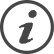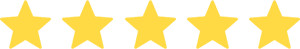### Excel Calculator: Cash Flow, NPV, ROI, IRR, WACC & Payback Period

#### 1 Excel sheet | 10 Powerpoint slides

Calculate in 3 minutes the key financials of your project or investment: Cash Flow, Cumulative Cash Flow, Net Present Value (NPV), ROI, Internal Rate of Return (IRR), Weighted Average Cost of Capital (WACC) & Payback Period. This Excel Calculator was created by ex-McKinsey & Deloitte Consultants and JP Morgan Investment Bankers. Don’t reinvent the wheel. Download Now.## \$299

Price seems too high?
Remember, the average daily rate of a McKinsey consultant is \$4,000. A lot more expensive than purchasing our Business & Consulting Toolkits. You get this for a fraction of this cost.

## Content Details

Expand all
I. A Excel Template:
1. Cells where you can easily input your initial investment, cash inflows & cash outflows
2. Multiple formulas that will automatically calculate Cash Flow, Cumulative Cash Flow, Net Present Value (NPV), ROI, Internal Rate of Return (IRR), Weighted Average Cost of Capital (WACC) & Payback Period
II. A tutorial providing:
1. A detailed definition of Cash Flow, Cumulative Cash Flow, Net Present Value (NPV), ROI, Internal Rate of Return (IRR), Weighted Average Cost of Capital (WACC) & Payback Period
2. A description of the formulas used